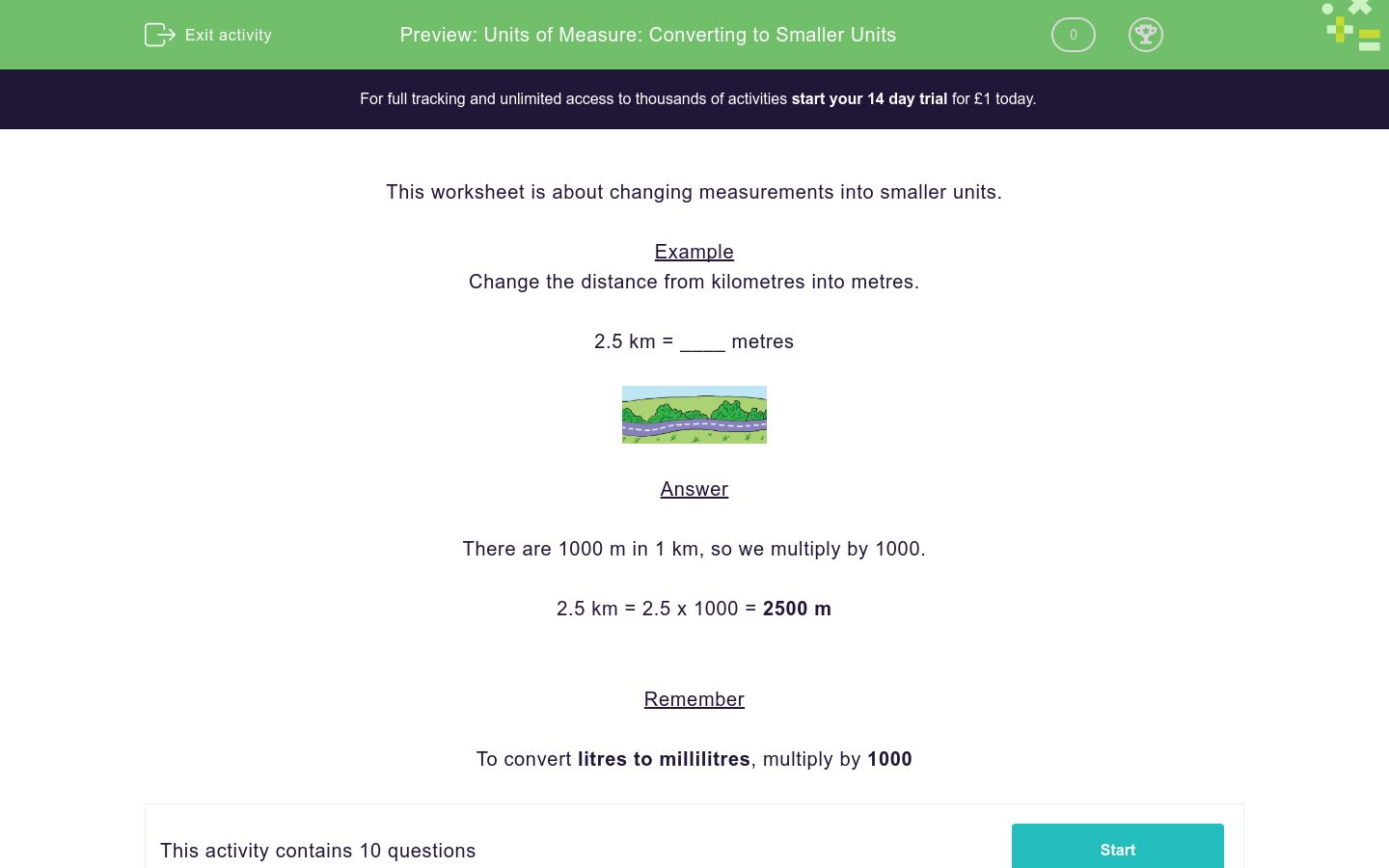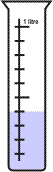# Units of Measure: Converting to Smaller Units

In this worksheet, students change the given measurements into smaller units.Key stage:  KS 2

Curriculum topic:  Measurement

Curriculum subtopic:  Convert Units of Measure

Difficulty level:### QUESTION 1 of 10

This worksheet is about changing measurements into smaller units.

Example

Change the distance from kilometres into metres.

2.5 km = ____ metresThere are 1000 m in 1 km, so we multiply by 1000.

2.5 km = 2.5 x 1000 = 2500 m

Remember

To convert litres to millilitres, multiply by 1000

Change the distance from kilometres into metres.

13 kilometres = ____ metresChange the distance from kilometres into metres.

10 kilometres = ____ metresChange the distance from kilometres into metres.

3 kilometres = ____ metresChange the distance from kilometres into metres.

9 kilometres = ____ metresChange the distance from kilometres into metres.

14 kilometres = ____ metresChange the measurement from  litres into millilitres.

6 litres = ____ millilitresChange the measurement from litres into millilitres.

2 litres = ____ millilitresChange the measurement from litres into millilitres.

10 litres = ____ millilitresChange the measurement from litres into millilitres.

14 litres = ____ millilitresChange the measurement from litres into millilitres.

4 litres = ____ millilitres• Question 1
Change the distance from kilometres into metres.

13 kilometres = ____ metres13000
• Question 2
Change the distance from kilometres into metres.

10 kilometres = ____ metres10000
• Question 3
Change the distance from kilometres into metres.

3 kilometres = ____ metres3000
• Question 4
Change the distance from kilometres into metres.

9 kilometres = ____ metres9000
• Question 5
Change the distance from kilometres into metres.

14 kilometres = ____ metres14000
• Question 6
Change the measurement from  litres into millilitres.

6 litres = ____ millilitres6000
• Question 7
Change the measurement from litres into millilitres.

2 litres = ____ millilitres2000
• Question 8
Change the measurement from litres into millilitres.

10 litres = ____ millilitres10000
• Question 9
Change the measurement from litres into millilitres.

14 litres = ____ millilitres14000
• Question 10
Change the measurement from litres into millilitres.

4 litres = ____ millilitres4000
---- OR ----

Sign up for a £1 trial so you can track and measure your child's progress on this activity.

### What is EdPlace?

We're your National Curriculum aligned online education content provider helping each child succeed in English, maths and science from year 1 to GCSE. With an EdPlace account you’ll be able to track and measure progress, helping each child achieve their best. We build confidence and attainment by personalising each child’s learning at a level that suits them.

Get startedStart your £1 trial today.
Subscribe from £10/month.